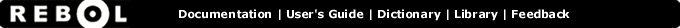# Not-equal? - Function Summary

## Summary:

Returns TRUE if the values are not equal.

## Usage:

not-equal? value1 value2

## Arguments:

value1 - The value1 argument.

value2 - The value2 argument.

## Description:

String-based datatypes are considered equal when they are identical or differ only by character casing (uppercase = lowercase).

 ``` print not-equal? "abc" "abcd" true```

 ``` print not-equal? [1 2 4] [1 2 3] true```

 ``` print not-equal? 12-sep-98 10:30 true```

## Related:

<> - Returns TRUE if the values are not equal.
= - Returns TRUE if the values are equal.
== - Returns TRUE if the values are equal and of the same datatype.
equal? - Returns TRUE if the values are equal.